## Math 501: Differential Geometry

Homework 5 <me@tylerlogic.com>

March 3, 2013
http://coursework.tylerlogic.com/courses/math501/homework05

1

Using the formula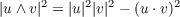we have the following sequence of equations for paramtrization x : U S for some regular surface S with V = x(U)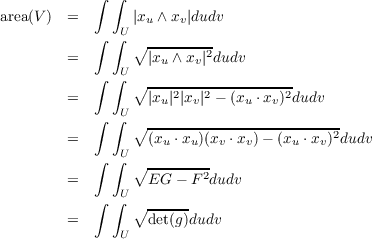where g =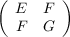2

Let S be the graph of a smooth function f : 2 with coordinate mapping X(u,v) = (u,v,f(u,v)). From this we get that Xu = (1,0,fu) and Xv = (0,1,fv).

(a) Coefficients of The First Fundamental Form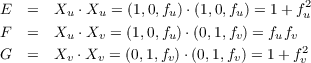(b) Length of α

Let α(t) be the curve in S with coordinate expression of (t,t) for 0 t 1, i.e. α(t) = (u(t),v(t)) where u(t) = t and v(t) = t. With this, the length of α is given by the following.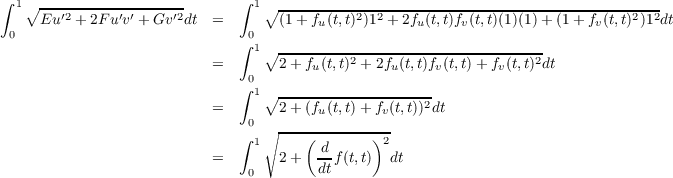(c) Area of V

Let V = X(U) for some bounded open set U of 2. Using the equation we derived in the first problem, we compute the area of V as follows.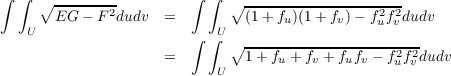3

Define the following parametrizations of the xy-plane in 3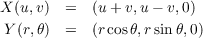We can then define the change of coordinate function, h : 2 2 by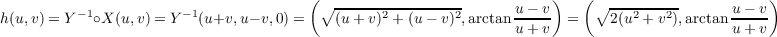with inverse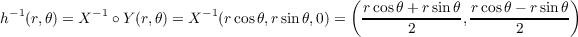resulting in the change-of-coordinate functions at the coordinate level of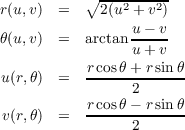Computing Xu = (1,1,0) and Xv = (1,-1,0) we can then compute Y r and Y θ in terms of Xu and Xv as follows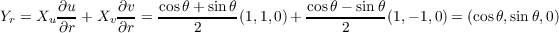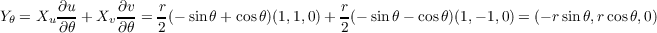4

The parametrization of the rotation of the regular plane curve, (f(v),g(v), of the xz-plane with x and z coordinates given by f(v) and g(v), respectively, is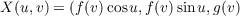(a)

With the above definition of the parametrization, we have the following differential of X.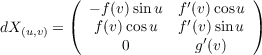Since the curve of rotation is a regular curve then g(v)0 for all v which tells us that the columns of dX(u,v) are linearly independent and therefore dX(u,v) is injective.

(b)

With the above definition of the parametrization we get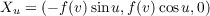and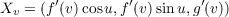from which we get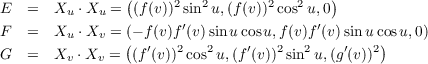5 do Carmo Page 109 problem 2

Let φ : S1 S2 be a local diffeomorphism with S2 orientable. Then there is some N2 : S2 3 that is a differentiable field of normal unit vectors. Hence N1 = N2 φ is differentiable in a neighborhood of any q S1, but since φ(q) S2, then N2(φ(q)), which is equal to N1(q), is a unit normal vector. Thus N2 : S1 3 is a differentiable field of unit normal vectors, i.e. S1 is orientable.